Web www.handwritingforkids.com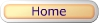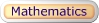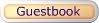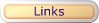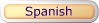### Subtraction - Worksheet Maker 2 Digits/1 Digit (Vertical)

This printable basic math subtraction worksheet generator will provides additional of math practice, boost test scores and prepare the child/children to meet the Grade 1, Grade 2, and Grade 3 curriculum standards.

This math worksheet maker will create a vertical math subtraction, 2 digits by 1 digit (2x1) equations with an option of "No Borrowing", "Borrowing", or "Mixed" (both borrowing and no borrowing) math equations, using the handwriting lines.. Note: This program will randomly select minuend numbers between 10-99, and subtrahend numbers between 0-9.

See sample math worksheets below:

 46 - 4 42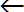minuendsubtrahenddifference

1. Select the number of math problems per page. (Default: 1 - 20)
2. Select Type of Math Problems. (Default: No Borrowing)
3. Click on submit to make your personalized vertical math subtraction worksheet.
4. Option: Page back and click Submit to create a different set of math problems.

Subtraction Worksheet Maker
2 Digits / 1 Digit (vertical)

 Enter the Number of Math Problems per page: Select a range 1 - 5 1 - 10 1 - 15 1 - 20 Default: 1 - 20 Type of Math Problems: Select a Type of Math No Borrowing Borrowing Mixed Default: (No Borrowing)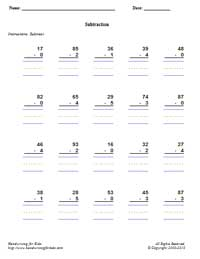Basic Handwriting for Kids Free for Non-Profit Use Ms. Readman, Rockford, IL Revised: